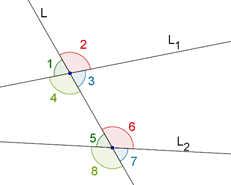# Transversals and Related Angles

Transversals and Related Angles
Go back to  'Angles'

For two or more lines, a transversal is any line which intersects two lines at distinct points. In the following figure, L1 and L2 are two lines which are cut at A and B by a transversal L0, resulting in a number of angles being formed:There is a specific terminology associated with the angles formed when a transversal cuts two lines, as shown above. Let us quickly go over that terminology by taking the example of the figure above:

### Corresponding angles

The following pairs of angles are corresponding angles:

• $$\angle 1$$ and $$\angle 5$$
• $$\angle 2$$ and $$\angle 6$$
• $$\angle 3$$ and $$\angle 7$$
• $$\angle4$$ and $$\angle 8$$

### Alternate interior angles

The following pairs of angles are alternate interior angles:

• $$\angle 3$$ and $$\angle 5$$
• $$\angle4$$ and $$\angle 6$$

### Alternate exterior angles

The following pairs of angles are alternate exterior angles:

• $$\angle 1$$ and $$\angle 7$$
• $$\angle 2$$ and $$\angle 8$$

### Co-interior angles

The following pairs of angles are co-interior angles:

• $$\angle 3$$ and $$\angle 6$$
• $$\angle4$$ and $$\angle 5$$

We will now go on to the specific case of two parallel lines being cut by a transversal.

Geometry
grade 9 | Questions Set 1
Geometry
Geometry
grade 9 | Questions Set 2
Geometry